# How to sort Dates by Month and Day Only in Excel

This post will guide you how to sort dates or birthdays by month or day only in Excel. How do I sort dates by Year or Month or Day only with a formula in Excel 2013/2016. How to use the MONTH function and DAY function to sort dates by month or day only in Excel.

## Sort Dates by Month

Assuming that you have a list of data in range A1:B5, which contain date values, and you need to sort those dates by month only. How to achieve it. You can use the MONTH function in combination with a helper column to achieve the result. Just do the following steps:

#1 type the following formula in the adjacent cell of Cell B1, then press Enter key to apply this formula.

`=MONTH(B1)`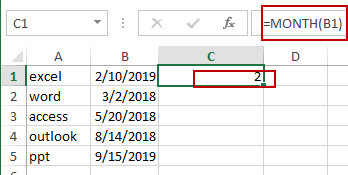#2 drag the AutoFill Handle over to other cells to apply this formula to get the month value from dates.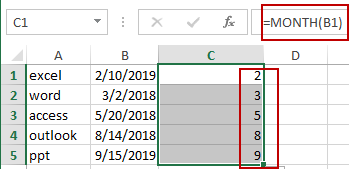#3 select all Month values in helper column. And go to DATA tab, click Sort Smallest to Largest or Sort Largest to Smallest button under Sort & Filter group. The Sort Warning dialog will open.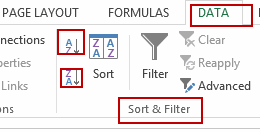#4 select Enable the selection radio button, and click Sort button. You will see that the dates have been sorted by month only. And you can now delete the helper column.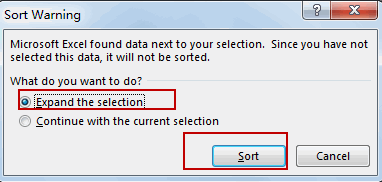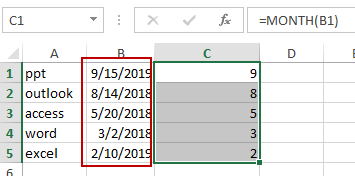## Sort Dates by Year

If you want to sort dates by Year only, you can repeat the above steps, just only use the following formula to instead of Month formula.

`=YEAR(B1)`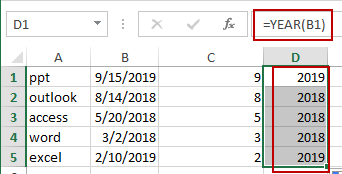## Sort Dates by Day

If you want to sort dates by Day only, you can also repeat the above steps, and just only use the following formula instead of the MONTH formula.

`=DAY(B1)`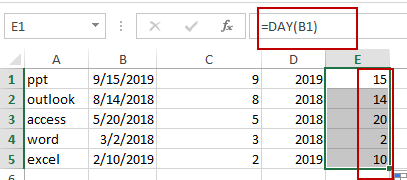Video: Sort Dates by Year/Month/Day

### Related Functions

• Excel YEAR function
The Excel YEAR function returns a four-digit year from a given date value, the year is returned as an integer ranging from 1900 to 9999. The syntax of the YEAR function is as below:=YEAR (serial_number)…
• Excel MONTH function
The Excel MONTH function returns the month of a date represented by a serial number. And the month is an integer number from 1 to 12. The syntax of the MONTH function is as below:=MONTH (serial_number)…
• Excel DAY function
The Excel DAY function returns a day of a date (from 1 to 31).The DAY function is a build-in function in Microsoft Excel and it is categorized as a DATE and TIME Function.The syntax of the DAY function is as below:= DAY (date_value)…